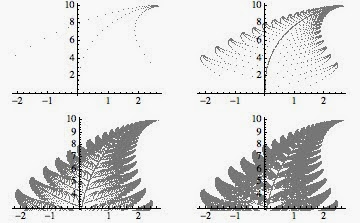Saturday, June 7, 2014

Fractals: a deterministic and recursive algorithm to reproduce it (Part II)

Abstract:Fern fractal estimation thru recursive and deterministic algorithm.

A fractal can be described as fixed point of a Markov process: given the proper contractive maps it's easy to generate it.
The question is: given the contractive maps, is it possible to generate the fractal using a pure deterministic approach?

Problem
We already observed that the iteration of the contractive maps starting from a fixed point that lyes on the border of the fractal returns points of the convex hull of the fractal.

• What's happen if we recursively apply such schema to the points obtained from the the above procedure?
• Is it possible recreate the markov process (or at least approximate it) removing any probabilistic aspects?
The below algorithm, given the contractive maps of the fractal, returns at each iteration better approximation of it.

At each iteration it considers the fractal as better approximation of the contour of the original image.

The Algorithm (by practical means)
1. Consider a fixed point $P_0$ of a contractive map $\tau_1$ that lyes on the convex hull of the fractal.
2. Choose randomly a point $P_i$ of the fractal and apply the above contractive map until the distance to $P_0$ is negligible.
3. Map the point calculated at step 2 using sequentially all the contractive maps.
4. Map each point obtained from point 3 the former step with $\tau_1$ till the distance to $P_0$ is negligible.
5. If[ITERATIONS< K]:
1. K--;
2. For each point obtained from point 4 go to point 3.
To explain how it works I plotted the list of points got using $K=1$ iterations of the algorithm:

Bigger is $K$, more accurate will be the result.
The above procedure works only if the contractive map $\tau_1$ has a fixed point that lyes on the convex hull of the fractal!!

Results:

I iterated the algorithm with $K=4$ times. At each iteration the algorithm returns a deeper description of the contour of the fractal (...even though definition of contour for a fractal doesn't make any sense, it gives at least a practical meaning):Results of the Recursive Algorithm iterated with K=1,2,3,4.

If we try to overlap the results obtained with the original fern we get:Original Fern, and the overlap with the results obtained using the recursive algorithm (K=4).
Conclusion and next steps
I showed how to depict the IFS generated thru the markovian process as a recursive and completely deterministic process.
We noticed also, that in the fractal there are special points (as for instance $P_0$) that play a crucial role to describe the IFS.

The question now is the following:
is it possible leverage such special points and the above recursive algorithm to estimate the parameters of the contractive maps?
... I will show you a procedure that partially answer the question and some other example of the algorithm above described!
Stay Tuned.
Cristian

1 comment:

1.Good Going bro :) nice blog.